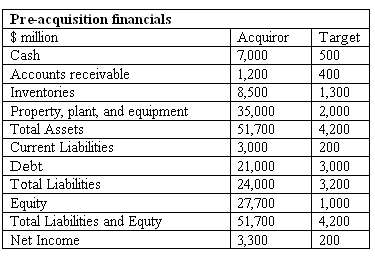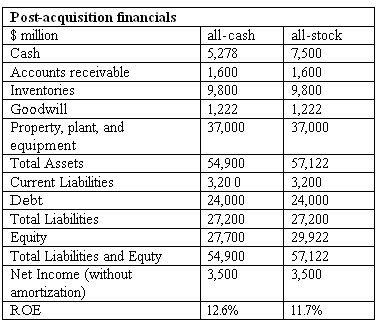### Seeing is believing!

Before you order, simply sign up for a free user account and in seconds you'll be experiencing the best in CFA exam preparation.

##### Subject 8. Accounting and International Considerations
If the clean surplus relationship holds, accounting policies should have no effect on the company's intrinsic value. Aggressive accounting increases book value at the expense of future earnings; conservative accounting constrains book value, but results in higher earnings in the future. This relationship holds only theoretically due to certain accounting issues.

1. Violations of clean surplus accounting.

Comprehensive income represents all changes in equity, except for those resulting from contributions from or distributions to the shareholders. It consists of net income and other comprehensive income. To accurately determine ROE for residual income model, we need to use the total comprehensive income as a measure of a company's earnings. Therefore, analysts need to adjust net income by certain items that are not reported on the income statement and not affect shareholders' equity directly.

• Fair value changes of some financial instruments.

2. Balance sheet adjustments for fair value.

To have a reliable measure of book value of equity, analysts need to ensure that all assets and liabilities are considered in valuation and fairly valued.

The first point addresses the need to account for off-balance-sheet assets and liabilities, such as operating leases, pension liabilities, take-or-pay and throughput arrangements, and special purpose vehicles involving sales of receivables. Some of these items affect the book value directly, others affect the ROE forecast.

The second point raises an issue of fair valuation of assets and liabilities. Due to the historical principle of accounting, some assets' book value may significantly depart from their fair market value. An analyst may need to adjust inventories, reserves and allowances, deferred tax assets and liabilities, and some other accounts to estimate the book value accurately.

3. Intangible assets.

Goodwill is the result of an acquisition in which the price paid for the target company is more than the value of the target's net assets. Therefore, goodwill cannot be internally generated. We explore the influence of goodwill on residual income model in the following example.

Example

An analyst is considering intrinsic value of two companies prior to and after the acquisition. Both companies distribute all income to the shareholders and have 9% required rate of return on equity.As the table shows, ROE of the acquiror is \$3,300/\$27,700 = 11.9%.
ROE of the target company is \$200/\$1,000 = 20%.

Since all income is distributed to the shareholders, neither of the companies is growing.

We first calculate the intrinsic value of the Acquiror: V0 = B0 + B0 x (ROE - r) / (r - g) = \$27,700 + \$27,700 x (0.119 - 0.09) / (0.09 - 0.00) = \$36,626.

Similarly, the value of the Target is: V0 = B0 + B0 x (ROE - r) / (r - g) = \$1,000 + \$1,000m x (0.20 - 0.09) / (0.09 - 0.00) = \$2,222.

The analyst assumes that the Acquiror purchases the Target for its fair value, \$2,222 and considers two payment options - 100% cash and 100% equity. He calculates the data for the post-acquisition balance sheet and income:The goodwill equals the difference between the deal consideration (\$2,222) and the Target's net assets (\$1,000). He calculates the value of the combined entity for both cases, using residual income model:

• All-cash: V0 = B0 + B0 (ROE - r) / (r - g) = \$27,700 + \$27,700 x (0.126 - 0.09) / (0.09 - 0.00) = \$38,888.
• All-stock: V0 = B0 + B0 x (ROE - r) / (r - g) = \$29,922 + \$29,922 x (0.117 - 0.09) / (0.09 - 0.00) = \$38,888.

In both cases the value of the combined entity equals the sum of pre-acquisition values, since the Target company was acquired for its fair value.

Finally, the analyst decides to calculate the value of the combined entity, assuming that goodwill is fully amortized using the straight-line method for 10 years. Disregarding the tax effect, amortization of goodwill decreases net income by \$1,222/10 = \$122. Taking goodwill amortization into account, ROE of the combined entity after all-cash acquisition is 12.2%.

This gives us the total post-acquisition value:

V0 = B0 + B0 x (ROE - r) / (r - g) = \$27,700 + \$27,700 x (0.122 - 0.09) / (0.09 - 0.00) = \$37,533, or \$1,355 lower than the intrinsic value without accounting for goodwill amortization. Since the acquiror paid the fair price for the target company, the combined entity's value should be equal to the total pre-acquisition value of the two companies.

Goodwill amortization is no longer amortized under both IFRS and U.S. GAAP.

Another intangible asset that needs particular attention when using residual income model is R&D costs. U.S. GAAP require expensing of essentially all R&D costs at the time when they are incurred. Capitalization and amortization of R&D costs is allowed under IAS. As a result, two identical companies based in Germany and the United States may have different patterns of net income and ROE. Since ROE is one of the drivers in the residual income model, analysts need to achieve uniformity of accounting principles applied to valuation to perform comparative analysis.

4. Nonrecurring items.

RI should be based on recurring items only. Nonrecurring items may result either from accounting rules, or from management decisions. Examples are:

• Unusual items.
• Extraordinary items.
• Restructuring charges.
• Discontinued operations.
• Accounting changes.

No adjustments to book value are necessary for these items, however, because nonrecurring gains and losses are reflected in the value of assets in place.

If such nonrecurring charges occur every year, they can be considered permanent and ROE should not be adjusted.

5. Aggressive accounting practices.

Some aggressive accounting practices may result in overstatement of assets or earnings, acceleration of revenues to the current period, or deferral of expenses to a later period. These activities increase earnings and book value. Normally, such items should be adjusted to achieve more conservative estimate of ROE and book value.

6. International considerations.

Accounting standards differ internationally and obviously accounting data needs to be adjusted accordingly. These differences may include the items that we have already discussed above:

• Violations of clean surplus accounting.
• Alternative treatment of intangibles.
• Different degree of accounting aggressiveness, etc.

The more such issues are present in the country's accounting standards, the less applicable is the residual income model.

User Comment
PASS0808 Not quite get it with "suppose instead continuing RI persistence factor is 0.4". What is that factor?
Rotigga V0 = B0 + Sum of Disc RI's + (RI(n+1) / (1 + r - w) / (1+r)^(n)
Where w = persistence factor
jamesleung is it something wrong here ?
should not it be 38,780 ??

All-cash: V0 = B0 + B0 (ROE - r) / (r - g) = \$27,700 + \$27,700 x (0.126 - 0.09) / (0.09 - 0.00) = \$38,888.